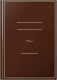# The Mathematical Theory of a Stationary QueueDefense Technical Information Center, 1967 - 16 pages
The case of a single channel is treated under the assumption that the sequence of waiting times is stationary (in mean neither increasing nor decreasing) and the service times have an arbitrary distribution (with characteristic function psi(xi)). The relative lengths P(k) of the time intervals with k customers waiting is determined by recurrence formulas. Formula (5) gives the mean waiting time gamma; mu k signifies here the k-th moment of the distribution of waiting times and alpha denotes the traffic intensity. The formula for phi(xi) gives an expression for the characteristic function for the waiting time distribution. Finally, formula (9) gives the variance of the waiting times.